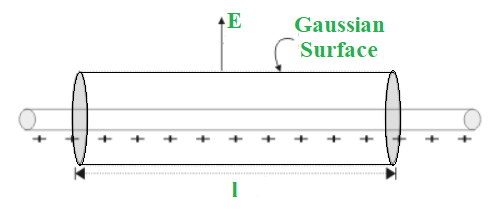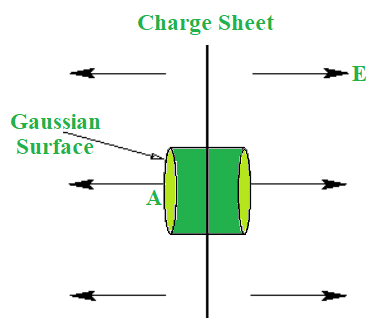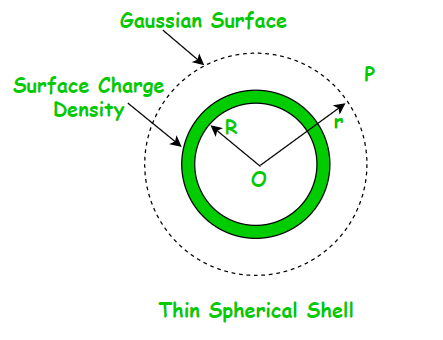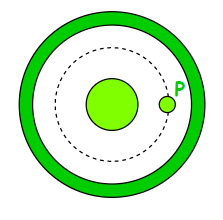Related Articles

# Applications of Gauss’s Law

• Last Updated : 05 Aug, 2021

According to Gauss’s Law, the total electric flux out of a closed surface equals the charge contained divided by the permittivity. The electric flux in an area is defined as the electric field multiplied by the surface area projected in a plane perpendicular to the field.

Now that we’ve established what Gauss law is, let’s look at how it’s used. Does it appear to you that this is already a challenging task? It’ll be a lot easier now. It’s worth noting that Gauss’s Law may be used to solve complicated electrostatic issues with unique symmetry such as planar, spherical, or cylindrical symmetry. So, contrary to popular belief, applying Gauss Law to your work might actually make it simpler!

### Applications of Gauss Law

We’ll look at a few of the applications of Gauss law right now. To begin with, we know that in some situations, calculating the electric field is fairly difficult and requires a lot of integration. Gauss’s Law is used to make calculating the electric field easier.

Let us first look at how we can apply the legislation before learning more about the applications. We need to pick a Gaussian surface that makes evaluating the electric field simple. To make things easier, one should employ symmetry. It’s also important to realize that the Gaussian surface does not have to match the real surface. It might be on either the inside or outside of the Gaussian surface.

### Electric Field due to Infinite Wire

Consider a wire that is infinitely long and has a linear charge density λ. To compute the electric field, we utilize a cylindrical Gaussian surface. The flux through the end of the surface will be 0 since the electric field E is radial.

Because the electric field and the area vector are perpendicular to each other, this is the case. We may argue that the electric field’s magnitude will be constant since it is perpendicular to every point on the curved surface.Electric Field due to Infinite Wire

The curved cylindrical surface has a surface area of 2 π r l. The electric flux flowing through the curve is equal to E × (2 π r l).

According to Gauss’s Law:

ϕ = q ⁄ ε0

E × (2 π r l) = λ l ⁄ ε0

E = λ ⁄ 2 π ε0 r

It’s important to note that if the linear charge density is positive, the electric field is radially outward. If the linear charge density is negative, however, it will be radially inward.

### Electric Field due to Infinite Plane Sheet

Consider an infinite plane sheet with a cross-sectional area A and a surface charge density σ. The infinite plane sheet is in the following position:Electric Field due to Infinite Plane Sheet

The electric field generated by an infinite charge sheet is perpendicular to the sheet’s plane. Consider a cylindrical Gaussian surface whose axis is perpendicular to the sheet’s plane. Gauss’s Law allows us to calculate the electric field E as follows:

ϕ = q ⁄ ε0

Charge q will be the charge density (σ) times the area (A) in continuous charge distribution. We shall only consider electric flow from the two ends of the imagined Gaussian surface when discussing net electric flux. We may explain it by the fact that the curved surface area and the electric field are perpendicular to each other, resulting in zero electric flux. As a result, the net electric flux:

ϕ = E A − (− E A)

ϕ = 2 E A

So,

2 E A = σ A ⁄ ε0

E = σ ⁄ 2 ε0

The above formula shows that the electric field generated by an infinite plane sheet is independent of the cross-sectional area A.

### Electric Field due to Thin Spherical Shell

Consider a thin spherical shell with a radius “R” and a surface charge density of σ. The shell possesses spherical symmetry. The electric field owing to the spherical shell can be calculated in two ways:

• Outside the spherical shell
• Inside the spherical shell

Let’s take a closer look at these two scenarios.Electric Field Outside the Spherical Shell

Take a point P outside the spherical shell at a distance r from the center of the spherical shell to get the electric field. We use a Gaussian spherical surface with radius r and center O for symmetry. Because all points are equally spaced “r” from the sphere’s center, the Gaussian surface will pass through P and experience a constant electric field E all around. So, Therefore, the total electric flux:

ϕ = q ⁄ ε0 = E × 4 π r2

The charge contained inside the surface, q = σ × 4 π R2. As a result,

E × 4 π r2 = σ × 4 π R2 ⁄ ε0

E = σ R2 ⁄ ε0 r2

The electric field can also be written in the form of charge as:

E = k q ⁄ r2

It’s important to keep in mind that if the surface charge density σ is negative, the electric field will be radially inward.

Electric Field Inside the Spherical Shell

Let’s look at a point P inside the spherical shell to see how the electric field there is. We may use symmetry to create a spherical Gaussian surface that passes through P, is centered at O, and has a radius of r. Now, based on Gauss’s Law,

ϕ = q ⁄ ε0 = E × 4 π r2

Since surface charge density is spread outside the surface, there is no charge contained inside the shell. Therefore, the electric field from the above formula is also zero, i.e.,

E = 0 since q = 0.

### Sample Problems

Problem 1: A hemispherical bowl of radius r is placed in a region of space with a uniform electric field E. Find out the electric flux through the bowl.

Solution:

The surafce area of the given bowl, dA = 2 π r2

The field lines are parallel the axis of the plane of the bowl,i.e., θ = 0°

The electric flux, ϕ = E (dA) cosθ

= E (2 π r2) cos0°

= E (2 π r2)

Hence, the electric flux through the bowl is E (2 π r2).

Problem 2: How does the electric flow via the Gaussian surface vary if the radius of the Gaussian surface containing a charge is halved?

Solution:

Even when the radius is half, the total charge contained by the Gaussian surface stays the same. As a result, according to Gauss’ theory, total electric flux remains constant. The electric flux will not vary as it passes through the Gaussian surface.

Problem 3: A cylindrical surface of radius r and length l, encloses a thin straight infinitely long conduction wire with charge density whose axis coincides with the surface. Find the formula for the electric flux through the cylinder’s surface.

Solution:

A thin straight infinitely long wire has a uniform linear charge distribution. Consider the charge enclosed by the cylindrical surface be q.

Linear charge density, λ = q ⁄ l

Therefore, charge enclosed by the surface, q = λ l

The total electric flux through the surface of cylinder, ϕ = q ⁄ ε0

= λ l ⁄ ε0

Hence, the formula for electric flux through the cylinder’s surface is λ l ⁄ ε0.

Problem 4: Why Gauss’s Law cannot be applied on an unbounded surface?

Solution:

Only a closed surface is valid for Gauss’s Law. The cube, whether solid or hollow, is a closed surface on which Gauss’s Law can be applied. However, because there is no meaning of charge confined by the surface in the case of an unbounded surface, Gauss’s Law cannot be applied.

Problem 5: A charge of 2×10-8 C is distributed uniformly on the surface of a sphere of radius 2 cm. It is covered by a concentric, hollow conducting sphere of radius 5 cm. Find the electric field at a point 3 cm away from the centre.

Solution:

Let us consider the below figure.Sphere inside a concentric shell.

Assume we need to locate the field at point P. P should be used to draw a concentric spherical surface. All of the points on this surface are comparable, and the field at each of them will be equal in magnitude and radial in direction due to symmetry.

The flux through this surface = ∮ E dS

= E ∮ dS

= E (4 π r2)

where r = 3 cm = 3 × 10-2 m

This flux is equal to the charge q contained within the surface divided by ε0 according to Gauss’ law.

Thus,

E (4 π r2) = q/ε0

E = q ⁄ 4 π ε0 r2

= ( 9 × 109) × [(2 × 10-8)/(9 × 10-4)]

= 2 × 105 N ⁄ C

The electric field at a point 3 cm away from the centre is 2 × 105 N ⁄ C.

Attention reader! All those who say programming isn’t for kids, just haven’t met the right mentors yet. Join the  Demo Class for First Step to Coding Course, specifically designed for students of class 8 to 12.

The students will get to learn more about the world of programming in these free classes which will definitely help them in making a wise career choice in the future.

My Personal Notes arrow_drop_up